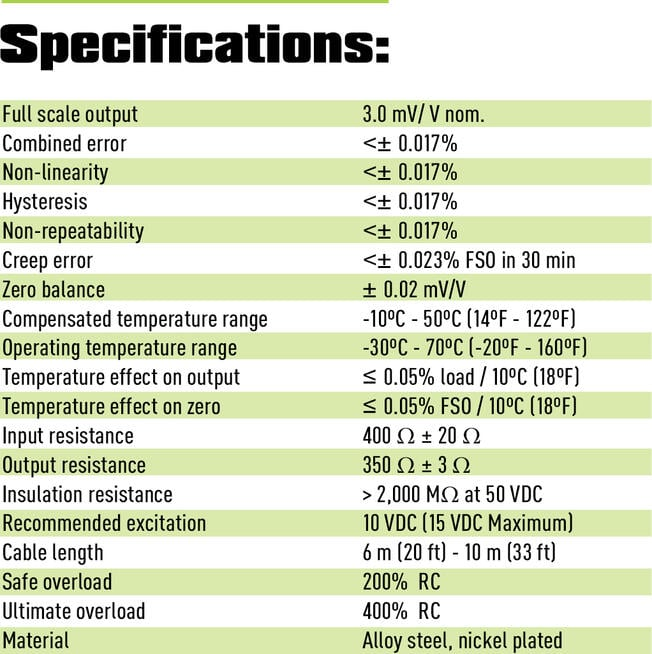# Load Cell Specifications & Datasheets

The specifications section of a load cell datasheet contains physical, electrical, and environmental information for your load cell.  The information listed will help you determine if the load cell in question is acceptable for your application.  Below is a sample load cell specification list as would be seen on a data sheet followed by explanations of each term used.Full scale output: The electronic output from a load cell. Measured at full scale. Units - mV/V

Combined error: The maximum deviation of the measured reading from the straight line drawn between readings at zero (no-load) and rated capacity as plotted on a graph. Measurements are taken as load both increases and decreases. Units - mV/V

Non-linearity: The maximum deviation of the calibration curve from a straight line drawn between the no-load and rated outputs; Measurements taken as load increases. Units - %FSO

Hysteresis: The maximum difference between multiple load cell output readings for the same load; one reading obtained by increasing the load from zero and the other by decreasing the load from full scale output; Final measurement taken at half rated output. Units - %FSO

Non-repeatability (repeatability):  The maximum difference between load cell output readings for repeated loadings of the same weight under identical environmental conditions. Units - %FSO

Zero balance (zero offset): The amount of deviation in output between true zero and a real load cell (at rated excitation) with zero load. Units - %FSO

Compensated temperature range: The temperature range in which a load cell is physically/electrically compensated to maintain rated output and zero balance (within limited specifications). Units - °F (°C)

Operating temperature range: The temperature extremes within which a load cell will operate without suffering permanent damage to functionality or accuracy. Units - °F (°C)

Temperature effect on output: The change in a load cell’s output due to a change in ambient temperature. Units - %FSO / °F (°C)

Temperature effect on zero: The change in zero balance due to a change in ambient temperature. Units - %FSO / °F (°C)

Input resistance: The total resistance of the load cell bridge circuit. Measured at zero load from the positive and negative excitation terminals with the output terminals open-circuited. Units - Ohms (Ω)

Output resistance: The total resistance of the load cell bridge circuit. Measured at zero load from the positive and negative signal terminals with the output terminals open-circuited. Units - Ohms (Ω)

Insulation resistance: The DC resistance measured between three points: the bridge circuit and the load cell element; the bridge circuit and the cable shield; the load cell element and the cable shield. Note: Typically measured at 50 VDC excitation. Units - Megaohms (MΩ)

Recommended excitation: The maximum excitation voltage required for a load cell to operate within its specifications. Units - VDC

Cable length: Standard length of cable supplied with the load cell with which the load cell is calibrated; changes in cable length affect calibration.

Safe overload: The maximum load which can be applied to a load cell without causing a permanent inability to perform within specifications. Units - %FS (% full scale)

Ultimate overload:  The maximum load which can be applied without causing structural failure.

Material: Component material of spring element.